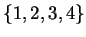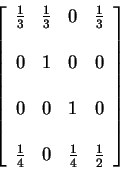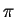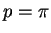STAT 380

Midterm Examination

Richard Lockhart 20 February 2002
=1=Instructions: This is an open book exam. You may use notes, books and a calculator. The exam is out of 25. Questions 1 and 3 are worth 5 marks each. Each of the 5 parts of question 2 is worth 3 marks. I will be marking for clarity of explanation as well as correctness. Without a clear explanation you should not expect to get more than half marks.

1.
A Markov Chain has state spaceand transition matrixIdentify all the communicating classes and say whether or not each is transient. [5 marks]

2.
Each day I get a random number of pieces of voice mail. I deal with, and delete, a random number of pieces of voice mail. When the mail box gets full any further messages received are lost. Here is a simplified model. Assume that my mail box can hold two messages. Each morning I get either 1 message or 0 messages. Each evening I delete either 1 message or 0 messages. The probability that I get 1 message is p regardless of what has happened in the past. The probability that I delete 1 message isif there is a message to delete. Let Xn be the number of messages on day n in the morning before any message arrives. Assume that X0=0; day 0 is the starting day.

(a)
Write out the transition matrix of the resulting Markov Chain. [3 marks]

(b)
Supposeand compute the probability that the mailbox is empty in the morning on day 2 (before the arrival of any mail). [3 marks]

(c)
Again supposing, show that in the long run the fraction of days on which I lose an email is p/3. [3 marks]

[NOTE: This question was wrong; you should just compute the correct fraction.]

(d)
Again supposing, in the long run what fraction of my e-mail will be lost? [3 marks]

(e)
Let T be the number of days till I lose my first e-mail and let mk be the expected value of T given that I start in state k (for k=0,1,2). Use first step analysis to derive a set of equations which would be solved to compute the mk. DO NOT SOLVE THE EQUATIONS. [3 marks]

3.
Imagine that buses arrive at a particular stop according to a Poisson process with rate 2 per hour. I start waiting at the stop at 1:00. Given that the second bus arrives between 2:00 and 3:00 what is the probability that the first bus arrived before 2:00? You may leave your answer as a formula--do not bother plugging the numbers into the calculator. [5 marks]

Extra space

Richard Lockhart
2002-02-26